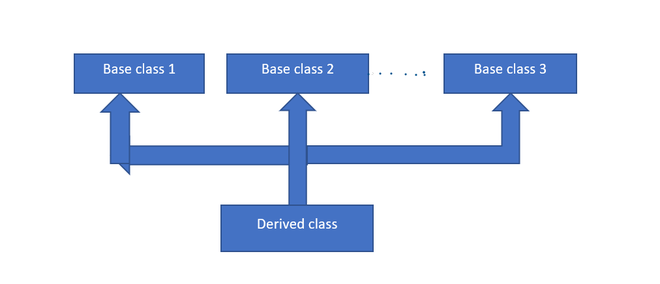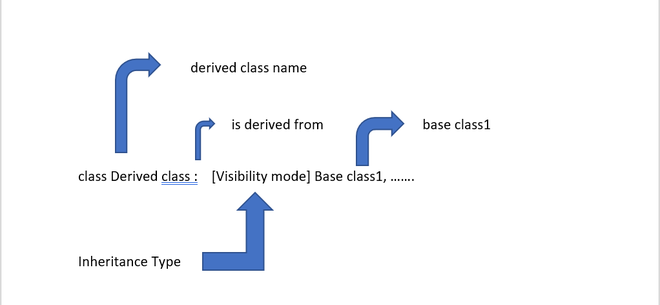GeeksforGeeks App
Open AppBrowser
Continue

# Constructor in Multiple Inheritance in C++

Constructor is a class member function with the same name as the class. The main job of the constructor is to allocate memory for class objects. Constructor is automatically called when the object is created.

Multiple Inheritance:

Multiple Inheritance is a feature of C++ where a class can derive from several(two or more) base classes. The constructors of inherited classes are called in the same order in which they are inherited.Multiple Inheritance Model

Syntax of Multiple Inheritance:Syntax of Multiple Inheritance:

class S: public A1, virtual A2
{
….
};

Here,
A2(): virtual base constructor
A1(): base constructor
S(): derived constructor

Example 1: Below is the C++ program to show the concept of Constructor in Multiple Inheritance.

## C++

 `// C++ program to implement``// constructor in multiple``// inheritance``#include``using` `namespace` `std;``class` `A1``{``  ``public``:``  ``A1()``  ``{``    ``cout << ``"Constructor of the base class A1 \n"``;``  ``}` `};` `class` `A2``{``  ``public``:``  ``A2()``  ``{``    ``cout << ``"Constructor of the base class A2 \n"``;``  ``}` `};` `class` `S: ``public` `A1, ``virtual` `A2``{``  ``public``:``  ``S(): A1(), A2()``  ``{``    ``cout << ``"Constructor of the derived class S \n"``;``  ``}``};` `// Driver code``int` `main()``{``  ``S obj;``  ``return` `0;``}`

Output

```Constructor of the base class A2
Constructor of the base class A1
Constructor of the derived class S ```

Time complexity: O(1)
Auxiliary Space: O(1)

Example 2: Below is the C++ program to show the concept of Constructor in Multiple Inheritance.

## C++

 `// C++ program to implement``// constructors in multiple``// inheritance``#include``using` `namespace` `std;``class` `A1``{``    ``public``:``        ``A1()``        ``{``            ``int` `a = 20, b = 35, c;``            ``c = a + b;``            ``cout << ``"Sum is:"` `<<``                     ``c << endl;``        ``}``};` `class` `A2``{``    ``public``:``        ``A2()``        ``{``            ``int` `x = 50, y = 42, z;``            ``z = x - y;``            ``cout << ``"Difference is:"` `<<``                     ``z << endl;``        ``}``};` `class` `S: ``public` `A1,``virtual` `A2``{``    ``public``:``        ``S(): A1(), A2()``        ``{``            ``int` `r = 40, s = 8, t;``            ``t = r * s;``            ``cout << ``"Product is:"` `<<``                     ``t << endl;``        ``}``};` `// Driver code``int` `main()``{``    ``S obj;``    ``return` `0;``}`

Output

```Difference is:8
Sum is:55
Product is:320```

Time complexity: O(1)
Auxiliary Space: O(1)

My Personal Notes arrow_drop_up